﻿ Harmonic Analysis of Tuning ForkExamples | Product | Murata Software Co., Ltd.Example15Harmonic Analysis of Tuning Fork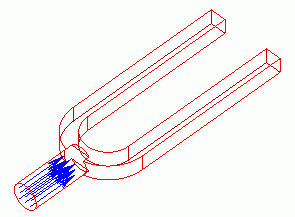General

• A tuning fork is forced to oscillate at certain frequencies.

• The deformation, the distributions of the displacement and the mechanical stress are solved.

• Unless specified in the list below, the default conditions will be applied.

Analysis Space

 Item Settings Analysis Space 3D Model unit m

Analysis Conditions

The analysis type is the harmonic analysis.

 Item Settings Solvers Mechanical Stress Analysis [Galileo] Analysis Type Harmonic analysis Options N/A

Analysis Condition Setting dialog box > Harmonic Analysis tab in > Set the frequencies of the forced oscillations as follows.

 Tab Item Settings Harmonic analysis Frequency Minimum: 500[Hz] Maximum: 510[Hz] Step Linear step by division number: Division number: 20

Model

The oscillations are forcibly applied on the bottom face of the knob of the turning fork. The oscillation amplitude and direction are set as boundary conditions.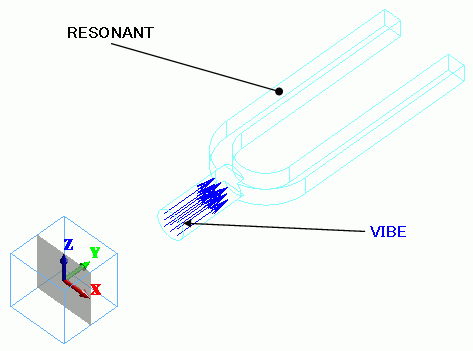Body Attributes and Materials

 Body Number/Type Body Attribute Name Material Name 8/Solid RESONANT 001_Al *

* Available from the Material DB

Boundary Conditions

In the harmonic analysis, the amplitude of the applied oscillation is set as the displacement amplitude in the boundary condition.

 Boundary Condition Name/Topology Tab Boundary Condition Type Settings Oscillation/Face Mechanical Displacement Select the Y component. UY=1×10^-5[m]

The oscillation is applied in the Y direction at the bottom face of the knob. The amplitude is 10[um].

Results

The displacement diagrams are shown below. The oscillation frequencies are 500[Hz], 505.5[Hz] and 510[Hz] respectively.

The contour diagram shows the displacement.

Displacements shown here are for a certain phase of the oscillation.

500[Hz]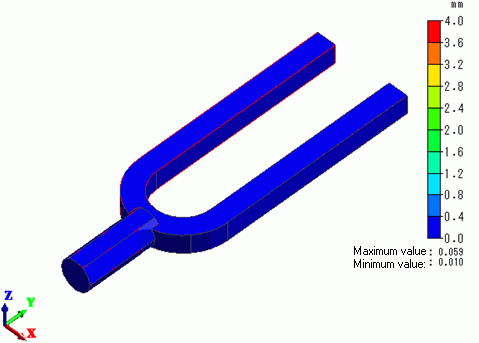505.5[Hz]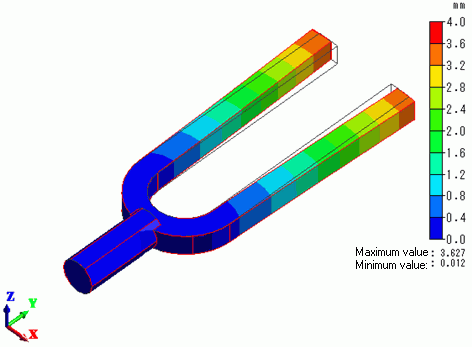510[Hz]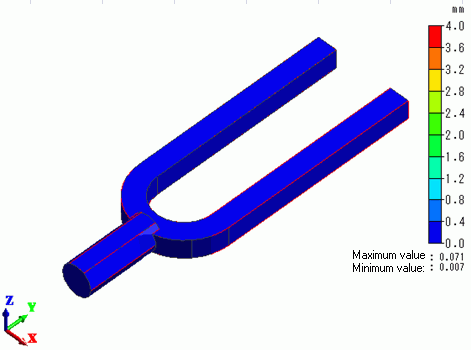The frequency characteristics of the displacement at the tips of the tuning fork is shown below.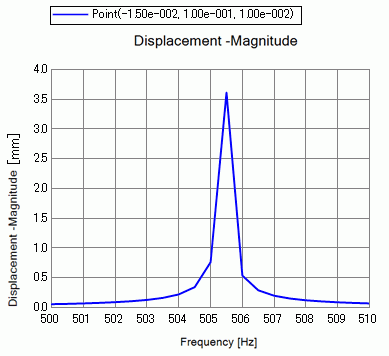The displacement becomes quite large when the applied frequency matches the resonant frequency of the tuning fork, which is around 519[Hz].

﻿